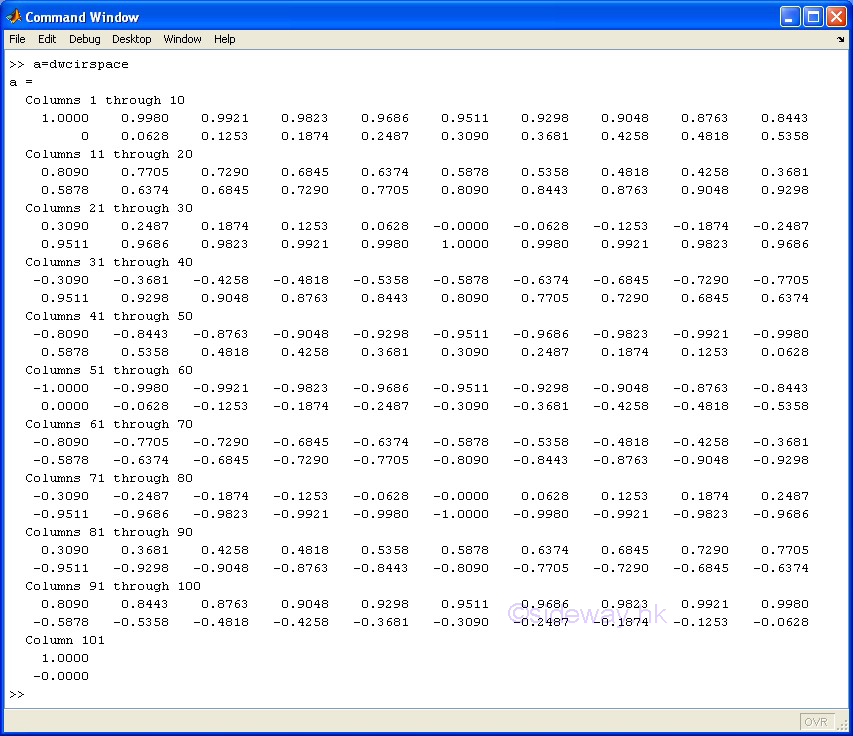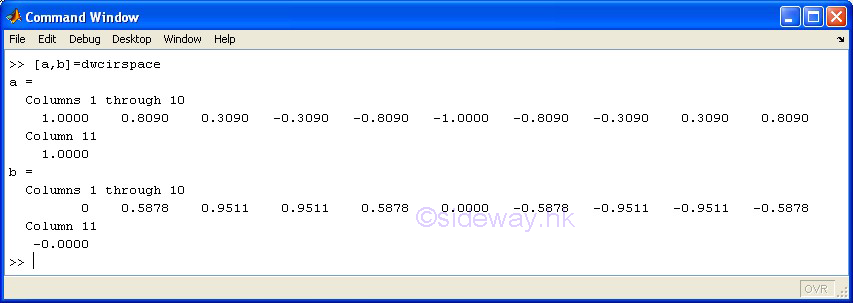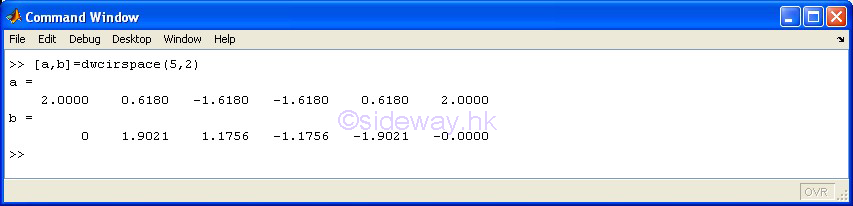output.to from Sideway

MatLabPari/gp

MatLabKnowledge Base

Draft for Information Only

# Content

```  MatLab: Frequently Used Codes   Array of Cyclic Points    dwcirspace    Example```

## MatLab: Frequently Used Codes

Major Reference Source: MatLab Verson 7.0

MatLab Package is designed for array or matrix operations, and therefore all data are stored in the form of arrays.

### Array of Cyclic Points

Circular spaced points is one of the common used form of points. Points are equally spaced in the form of a circle.

#### dwcirspace

Code:

function varargout=dwcirspace(varargin)
%dwcirspace creates a matrix of cyclic or circular spaced points
%
%varargout: (a,[b])
%
%varargint: ([a],[b],[c],[d])
%
% dwcirspace
% construct an 2x101 matrix of 101 circular spaced points with circumcircle centre at [0,0] and circumcircle radius equals to 1.
%
% dwcirspace(n)
% for n=1 an 2x1 vector of a single point is created at y equals to 0 and x equals to 0 plus offset radius 1 based on circumcircle centre at [0,0].
% for n=2 an 2x2 matrix of two points for a line is created parallel to x-axis at y equals to 0 and x equals to 0 plus offset radius 1 based on circumcircle centre at [0,0].
% for n>=3 an 2xn+1 matrix of n+1 circular spaced points is created
% construct an 2xn+1 matrix of n+1 circular spaced points with circumcircle centre at [0,0] and circumcircle radius equals to 1.
%
% dwcirspace(n,r)
% construct an 2xn+1 matrix of n+1 circular spaced points with circumcircle centre at [0,0] and circumcircle radius equals to r.
%
% dwcirspace(n,r,x)
% construct an 2xn+1 matrix of n+1 circular spaced points with circumcircle centre at [x,0] and circumcircle radius equals to r.
%
% dwcirspace(n,r,x,y)
% construct an 2xn+1 matrix of n+1 circular spaced points with circumcircle centre at [x,y] and circumcircle radius equals to r.
%
% ---------
% Copyright (c) 2014, All Right Reserved, http://sideway.hk/
% LastChanged:20/06/2014
% version:000.00001 created 20/06/2014 from sideway.hk
%
%
if nargin==0
n=100;r=1;x=0;y=0;
elseif nargin==1
n=varargin{1};r=1;x=0;y=0;
elseif nargin==2
n=varargin{1};r=varargin{2};x=0;y=0;
elseif nargin==3
n=varargin{1};r=varargin{2};x=varargin{3};y=0;
elseif nargin==4
n=varargin{1};r=varargin{2};x=varargin{3};y=varargin{4};
else
error('in argument more than four');
end
if n<=0
error('number of point must be greater than zero');
end
th=(0:1/n:1)*2*pi;
xunit=r*cos(th)+x;
yunit=r*sin(th)+y;
if n==1
xunit(2)=[];
yunit(2)=[];
elseif n==2
xunit(3)=[];
yunit(3)=[];
end
if nargout==0
error('out argument less than one');
elseif nargout==1
vrargout{1}=[xunit; yunit];
elseif nargout==2
varargout{1}=xunit;
varargout{2}=yunit;
else
error('out argument more than two');
end

#### Example

• Use function dwcirspace with default value to generate a 2x11 matrix

Example:• Use function dwcirspace with default value to generate two 1x11 vectors

Example:• Use function dwcirspace with repeating number n equals to 5 and radius r equals to 2 to generate two 1x6 vectors

Example:•

ID: 140600010 Last Updated: 6/20/2014 Revision: 0Home 5

Management

HBR 3

Information

Recreation

Culture

Chinese 1097

English 339

Computer

Hardware 249

Software

Application 213

Latex 52

Manim 205

KB 1

Numeric 19

Programming

Web 289

Unicode 504

HTML 66

CSS 65

SVG 46

ASP.NET 270

OS 429

Python 72

Knowledge

Mathematics

Algebra 84

Geometry 34

Calculus 67

Engineering

Mechanical

Rigid Bodies

Statics 92

Dynamics 37

Control

Natural Sciences

Electric 27